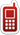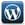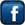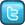MLK Home & Online Tutor+91-836-801-8076+91-999-034-6833Home Tutors available in Ghaziabad, Noida & East Delhi | Ghaziabad- Indirapuram, Vaishali, Vasundhara, Kaushambhi, Shahibabad, Shalimar Garden, Siddharth Vihar, Pratap Vihar, Crossing Republik, Mohan Nagar, Kavi Nagar, Raj Nagar, Shastri Nagar, Nehru Nagar, Patel Nagar, Model Town, Govind Puram, Chiranjeev Vihar, Gandhi Nagar, Lal Kuan, Wave City, Raj Nagar Extension | Noida- Noida(All Sectors), Greater Noida, Noida West(Noida Extension-Gour City-1,2; Amarpali Dream Valley; SuperTech Eco Village) | Delhi- East Delhi(Preet Vihar, Vivek Vihar, Shahdara, Mayur Vihar, Patparganj, Lakshmi Nagar, Shakarpur), South Delhi, West Delhi, North Delhi & Central Delhi.

## AREA WISE CHEMISTRY TUTORSFollow @tutormlk# Math Tutoring for Grade 8- Find Math home Tutors, Online Tutors, home Tuition & Private Tutors for Class VIII

Is your child struggling in school? Does your child stuck on math homework?

Or

Are you having difficulty working out math problems? Are you looking out for a grade 8 math home tutor?

MLK Home & Online Tutor can help you find the most qualified home & Online Math tutors for class/grade 8 across Ghaziabad, Noida, Delhi, Bangalore in Karnataka, Pune in Maharashtra, Hyderabad in Andhra Pradesh, Mumbai in Maharashtra, New Delhi in Delhi, Gurgaon in Haryana, Chandigarh in Punjab and Haryana, Noida in Uttar Pradesh, Ahmedabad in Gujarat & Chennai in Tamilnadu.

MLK Home & Online Tutor can help you find Maths tutors for class/grade VIII we have available near you. We will send him to your home for one free trial class, if satisfied with his tutoring, then continue. We are the only place, where just a single Call or Message will recommend you Maths tutor class/grade 8 for your personal needs, arranged according to their rates and distance from you. Maths can be an extremely tough subject to get to grips with, but private Maths tuition can really help your learning!

If you are a class/grade VII Maths tutor wishing to provide home tutoring in Maths class/grade 8, or in any of our other subjects, please register with MLK Home & Online Tutor.

Click here for a Home Tutor or an Online Tutor? MLK Home & Online Tutoring Service will assist your child in simplifying concepts and building confidence in approaching math. We serve students from Grade 6-12. In addition to math, tutoring is available in all core subjects-Physics, Chemistry, General science, Social studies test preparation including OLYNPIADS, NTSE exams, SAT, GMAT, GRE, MAT, CAT and foundation course for AIEEE and IIT-JEE.

## Get the Math Help Now!

Getting help is simple. You just tell us what you are working on and we match a professional Home tutor. Our expert, private, professional home tutor helps you solve your math problems step by step until your home work is finished. Home tutor at MLK Home and Online Tutoring Service provides you with worksheet and problem sets to practice your skills.

## Get a Home Tutor for Math Now!

Our home tutor is trained to identify the exact obstacle that may be hindering students' studies. He supplements the course's curriculum to ensure efficient and motivating home tutoring. Our home tutors are flexible in their schedule as they are available seven days a week for In-home tutoring.

## Affordable Math Test Preperation Programs

MLK Home and Online Tutoring Service 's Math Refresher course is designed to build a strong foundation in the basic math skills essential for success on the following tests:

 SAT GMAT GRE CAT MAT IIT-JEE AIEEE OLYMPIADS NDA SSC Bank PO/Clerk RRB Delhi & UP-SI Exam

## Topics Covered by our Math Home Tutoring Program:

 Arithmetic Pre-Algebra Algebra 1 Algebra 2 Linear Algebra Geometry Pre-Calculus Calculus Statistics & Probability Trigonometry General Math

## Number System

### (i) Rational Numbers

• Properties of rational numbers. (including identities). Using general form of expression to describe properties
• Consolidation of operations on rational numbers.
• Representation of rational numbers on the number line
• Between any two rational numbers there lies another rational number (Making children see that if we take two rational numbers then unlike for whole numbers, in this case you can keep finding more and more numbers that lie between them.)
• Word problem (higher logic, two operations, including ideas like area)

### (ii) Powers

• Integers as exponents.
• Laws of exponents with integral Powers

### (iii) Squares, Square roots, Cubes, Cube roots.

• Square and Square roots
• Square roots using factor method and division method for numbers containing (a) no more than total 4 digits and (b) no more than 2 decimal places
• Cubes and cubes roots (only factor method for numbers containing at most 3 digits)
• Estimating square roots and cube roots. Learning the process of moving nearer to the required number.

### (iv) Playing with numbers

• Writing and understanding a 2 and 3 digit number in generalized form (100a + 10b + c , where a, b, c can be only digit 0-9) and engaging with various puzzles concerning this. (Like finding the missing numerals represented by alphabets in sums involving any of the four operations.) Children to solve and create problems and puzzles.
• Number puzzles and games
• Deducing the divisibility test rules of 2, 3, 5, 9, 10 for a two or three-digit number expressed in the general form.

## Algebra

### (i) Algebraic Expressions

• Multiplication and division of algebraic exp.(Coefficient should be integers)
• Some common errors (e.g. 2 + x ≠ 2x, 7x + y ≠ 7xy )
• Identities (a ± b)2 = a2 ± 2ab + b2, a2 – b2 = (a – b) (a + b) Factorisation (simple cases only) as examples the following types a(x + y), (x ± y)2, a2 – b2, (x + a).(x + b)
• Solving linear equations in one variable in contextual problems involving multiplication and division (word problems) (avoid complex coefficient in the equations)

## Ratio and Proportion

• Slightly advanced problems involving applications on percentages, profit & loss, overhead expenses, Discount, tax.
• Difference between simple and compound interest (compounded yearly up to 3 years or half-yearly up to 3 steps only), Arriving at the formula for compound interest through patterns and using it for simple problems.
• Direct variation – Simple and direct word problems
• Inverse variation – Simple and direct word problems
• Time & work problems– Simple and direct word problems

## Geometry

### (i) Understanding shapes:

• Properties of quadrilaterals – Sum of angles of a quadrilateral is equal to 3600 (By verification)
• Properties of parallelogram (By verification)
• (i) Opposite sides of a parallelogram are equal,
• (ii) Opposite angles of a parallelogram are equal,
• (iii) Diagonals of a parallelogram bisect each other. [Why (iv), (v) and (vi) follow from (ii)]
• (iv) Diagonals of a rectangle are equal and bisect each other.
• (v) Diagonals of a rhombus bisect each other at right angles.
• (vi) Diagonals of a square are equal and bisect each other at right angles.

### (ii) Representing 3-D in 2-D

• Identify and Match pictures with objects [more complicated e.g. nested, joint 2-D and 3-D shapes (not more than 2)].
• Drawing 2-D representation of 3-D objects (Continued and extended)
• Counting vertices, edges & faces & verifying Euler’s relation for 3-D figures with flat faces (cubes, cuboids, tetrahedrons, prisms and pyramids)

### (iii) Construction:

• Given four sides and one diagonal
• Three sides and two diagonals
• Three sides and two included angles
• Two adjacent sides and three Angles

#### Mensuration

• Area of a trapezium and a polygon.
• Concept of volume, measurement of volume using a basic unit, volume of a cube, cuboid and cylinder
• Volume and capacity (measurement of capacity)
• Surface area of a cube, cuboid, cylinder.

## Data handling

• Reading bar-graphs, ungrouped data, arranging it into groups, representation of grouped data through bar-graphs, constructing and interpreting bar-graphs.
• Simple Pie charts with reasonable data numbers
• Consolidating and generalising the notion of chance in events like tossing coins, dice etc. Relating it to chance in life events. Visual representation of frequency outcomes of repeated throws of the same kind of coins or dice. Throwing a large number of identical dice/coins together and aggregating the result of the throws to get large number of individual events. Observing the aggregating numbers over a large number of repeated events. Comparing with the data for a coin. Observing strings of throws, notion of randomness

## Introduction to graphs

### Preliminaries:

• Axes (Same units), Cartesian Plane
• Plotting points for different kind of situations (perimeter vs length for squares, area as a function of side of a square, plotting of multiples of different numbers, simple interest vs number of years etc.)Follow @tutormlkFollow @tutormlk